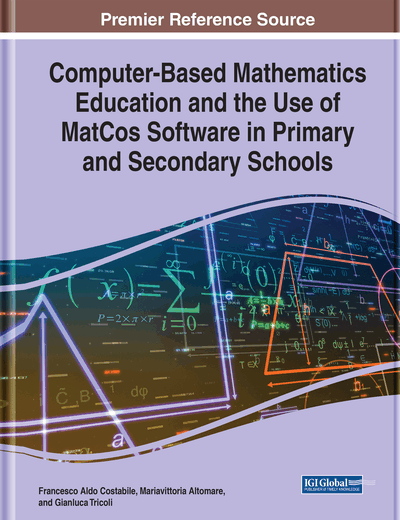# Activities in the Secondary School: An Arithmetic, Algebraic, Analytic path

DOI: 10.4018/978-1-7998-5718-1.ch008
OnDemand:
(Individual Chapters)
Available
\$37.50
No Current Special Offers

## Abstract

In this chapter there is the presentation of a vertical path on the main topics of arithmetic-algebra-infinitesimal calculus and numerical methods, which are an object of study in the secondary school. Naturally, the attention will be focused on the “virtual” phase, that is the applications with the computer and the MatCos 3.X environment, both as graphical-numerical experimentation, of intuitive support to the understanding of the concepts, that as a necessary moment for the actual calculation in the applications. It presents a TLS based on a real problem, from which the whole presented methodology shines through: from problem solving, to mathematical and numerical modeling, to the formulation of the solving algorithm and its implementation in the MatCos 3.X environment.
Chapter Preview

... disregard for application and intuition leads to isolation and atrophy of mathematics … ~R. Courant, F. John (1965)

Top

## 2. Arithmetic

The concept of natural number and the related operations with execution algorithms are considered as having been acquired at the primary school, except for some reminders and further explanation where necessary, which the teacher will undertake. Just to test memories or see what has really been internalized, the teacher in the secondary school, at least in the first days of the school year, can resort to the playful aspect of Mathematics, to arouse interest and not indifference in young students. On the other hand, the high pedagogical value that can be recognized from the playful aspect has been reported several times (Gardner, 1987) (Ghersi, 1996) (Pappas, 1995). An example of this is the magic square.

## Key Terms in this Chapter

Numerical Method: Is a mathematical tool designed to solve numerical problems. The implementation of a numerical method with an appropriate convergence check in a programming language is called a numerical algorithm.

MatCos 3.X: Programming environment oriented towards mathematics, aimed at high school.

Mathematic Modelling: Is the art of translating problems from an application area into tractable mathematical formulations whose theoretical and numerical analysis provides insight, answers, and guidance useful for the originating application.

Calculus: Is the mathematical study of continuous change, in the same way that geometry is the study of shape and algebra is the study of generalizations of arithmetic operations.

Arithmetic: Branch of mathematics in which numbers, relations among numbers, and observations on numbers are studied and used to solve problems.

## Complete Chapter List

Search this Book:
Reset